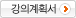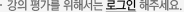## 주메뉴

### 디지털제어시스템

• 경북대학교
• 김민영
• 주제분류
공학 >전기ㆍ전자 >전자공학
• 강의학기
2016년 1학기
• 조회수
4,419
•
강의계획서#### 차시별 강의1.Introduction to digital control systems Introduction to digital control systems2.Sampling and reconstruction Sampling and reconstructionSampling and reconstruction Sampling and reconstruction3.Z-transforms: definition, properties and theorems Z-transforms: definition, properties and theoremsZ-transforms: definition, properties and theorems Z-transforms: definition, properties and theorems4.Sampled Data Systems (1) Sampled Data Systems (1)5.Sampled Data Systems (2) Sampled Data Systems (2)6.Discrete Equivalents (1) Discrete Equivalents (1)Discrete Equivalents (1) Discrete Equivalents (1)7.Discrete Equivalents (2) Discrete Equivalents (2)8.Midterm Midterm9.Design using Tranformation Technique-Classical Method (1) Design using Tranformation Technique-Classical Method (1)10.Design using Tranformation Technique-Classical Method (2) Design using Tranformation Technique-Classical Method (2)11.STATE-SPACE Analysis STATE-SPACE AnalysisSTATE-SPACE Analysis STATE-SPACE Analysis12.State Space Analysis. Problems State Space Analysis. Problems13.State-Space Analysis State-Space Analysis14.State-Space Analysis (cont) State-Space Analysis (cont)#### 연관 자료#### 사용자 의견#### 이용방법

• 문서 자료 이용시 필요한 프로그램 [바로가기]

※ 강의별로 교수님의 사정에 따라 전체 차시 중 일부 차시만 공개되는 경우가 있으니 양해 부탁드립니다.